Next: Worked Examples Up: Electric Current Previous: Energy in DC Circuits

## Power and Internal Resistance

Consider a simple circuit in which a battery of emf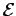and internal resistancedrives a current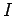through an external resistor of resistance(see Fig. 17). The external resistor is usually referred to as the load resistor. It could stand for either an electric light, an electric heating element, or, maybe, an electric motor. The basic purpose of the circuit is to transfer energy from the battery to the load, where it actually does something useful for us (e.g., lighting a light bulb, or lifting a weight). Let us see to what extent the internal resistance of the battery interferes with this process.

The equivalent resistance of the circuit is(since the load resistance is in series with the internal resistance), so the current flowing in the circuit is given by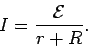(145)

The power output of the emf is simply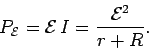(146)

The power dissipated as heat by the internal resistance of the battery is(147)

Likewise, the power transferred to the load is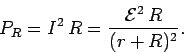(148)

Note that(149)

Thus, some of the power output of the battery is immediately lost as heat dissipated by the internal resistance of the battery. The remainder is transmitted to the load.

Letand. It follows from Eq. (148) that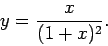(150)

The functionincreases monotonically from zero for increasingin the range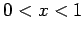, attains a maximum value ofat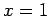, and then decreases monotonically with increasingin the range. In other words, if the load resistanceis varied at constantandthen the transferred power attains a maximum value of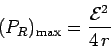(151)

when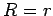. This is a very important result in electrical engineering. Power transfer between a voltage source and an external load is at its most efficient when the resistance of the load matches the internal resistance of the voltage source. If the load resistance is too low then most of the power output of the voltage source is dissipated as heat inside the source itself. If the load resistance is too high then the current which flows in the circuit is too low to transfer energy to the load at an appreciable rate. Note that in the optimum case,, only half of the power output of the voltage source is transmitted to the load. The other half is dissipated as heat inside the source. Incidentally, electrical engineers call the process by which the resistance of a load is matched to that of the power supply impedance matching (impedance is just a fancy name for resistance).Next: Worked Examples Up: Electric Current Previous: Energy in DC Circuits
Richard Fitzpatrick 2007-07-14